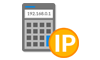## Determining Hosts Available

##### How to determine the number of hosts possible in a subnet:
If we want to determine the numbers of hosts in the subnet, we count the number of zeros in the host octet(s) of the subnet mask.

If we are provided the network address written in CIDR as 192.168.5.0/20, we would write out the prefix in binary form as below, where “n” is a network bit and “h” is a host bit.

```                    1 1 1 1 1 1 1 1 . 1 1 1 1 1 1 1 1 . 1 1 1 1 0 0 0 0 . 0 0 0 0 0 0 0 0
n n n n n n n n . n n n n n n n n . n n n n h h h h . h h h h h h h h
```

To figure out the number of hosts in each subnet, we count the number of host bits left over (the zeros). In the example above, we have 12 zeros in the subnet mask for the host bits.

The formula to use is below, where h = the number of host bits:

(2h) – 2

We subtract two because two host IPs are always reserved, the very first address in the subnet for the network address and the very last address in the subnet or the broadcast address.

In our example, this would be:

(212) – 2 = (4096 – 2) = 4094 usable address in a /20 subnet.